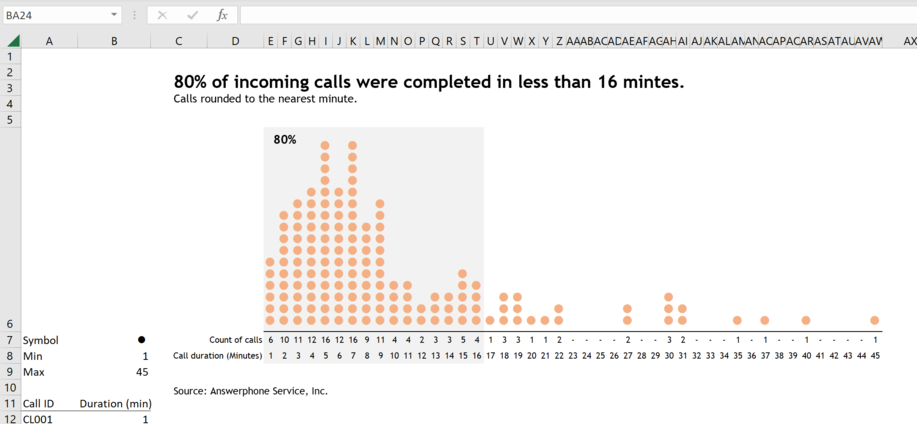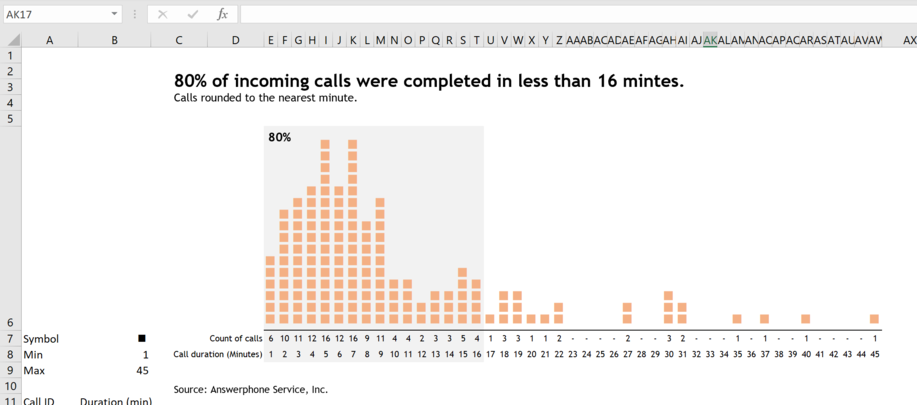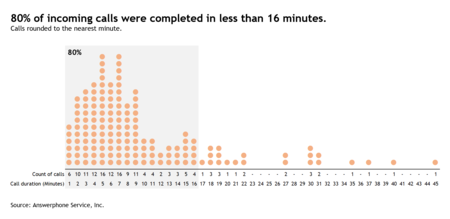• WORK
• TOOLS
• TRAINING
• VIEWS
• CONTACT

Views > Excel charts > How to create a histodot plot in Excel

Dot plots come in different variations.

First, we have the William Cleveland Dot Plot which is similar to a bar graph but uses position to encode data rather than height or length.

Second, we have the Unit charts which are also similar to bar charts—in the sense that they use length/height to encode data—but display each unit of measure as a stack of single mark or symbol instead of a continuous bar.

Third, there is the Wilkinson Dot Plot, which is a histogram where individual observations are displayed—using a dot or other symbol—on a continuous scale.

Finally, we have the Histodot plot—a name coined by Leland Wilkinson—to differentiate it from its more versatile sibling the Wilkinson Dot Plot. The difference between the two plots is that the latter displays the data on a continuous scale while the former uses simple histogram binning instead.

The graph below is a Histodot plot. It shows the distribution of the time required in minutes to complete various incoming calls. The data collected includes 150 random calls that were monitored and timed. The calls are rounded to the nearest minute.

1. I’ll start by defining the symbol     that I want to use in the chart. To do that, select cell B7, and on the Insert tab click on Symbol in the Symbols group.
2. In the Symbol dialog box select Wingdings from the Font dropdown list.
3. Scroll down through the matrix of icons and select the    icon as per the screenshot below. Click the Insert button. Next press the Close button to close the Symbol dialog box.

4. Press the Enter key on the keyboard to enter the symbol in cell B7.
5. Add the text label "Symbol" in cell A7. The Excel sheet should like this:

13. In cell E6 type the following formula:
= REPT(B7,E7#)
14. Select the range E6:AW6 and change the font to Wingdings, its size to 14 and its color to Orange. The Excel sheet should look like this:

# HOW TO CREATE A HISTODOT PLOT IN EXCEL

HOW TO CREATE A HISTODOT PLOT IN EXCEL

15. On the worksheet select the range E6:AW6. Right-click the selected range and then, on the shortcut menu, click Format Cells.
16. In the Format Cells dialog box, on the Alignment tab, change the Text Orientation to 90 degrees as per below screenshot.

21. Select the range E6:T8 and set the cells Fill Color to light grey.
22. Select the range E6:AW6 and apply the Thick Bottom Border to add a horizontal line.
23. Add title, source and annotation.
The worksheet should look like this:

24. Change the symbol in cell B7 and see how the chart adapts. Here is a proposal with squares instead of circles: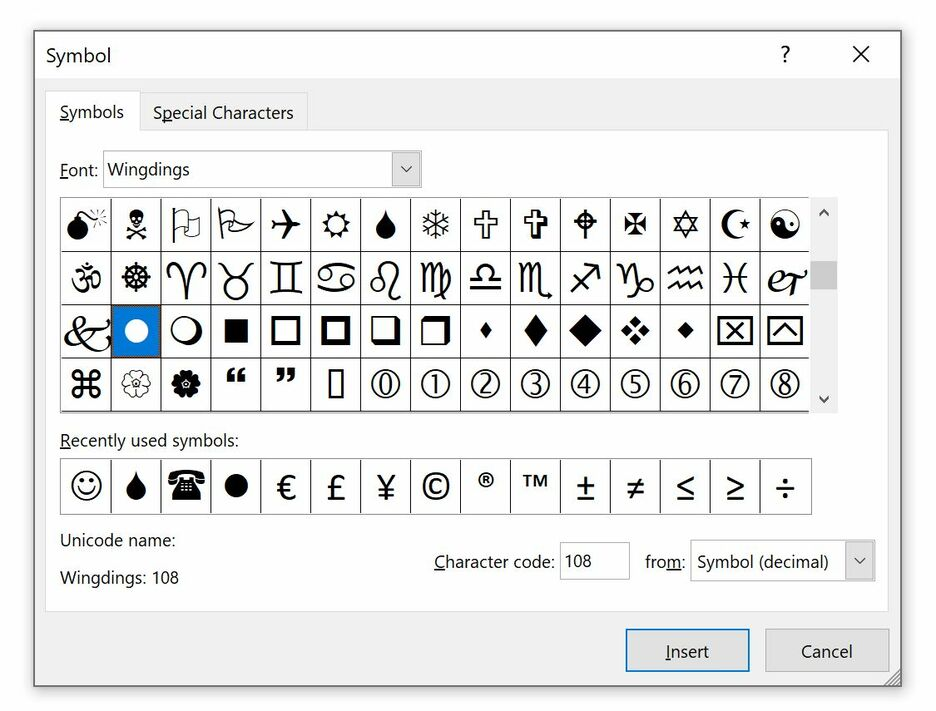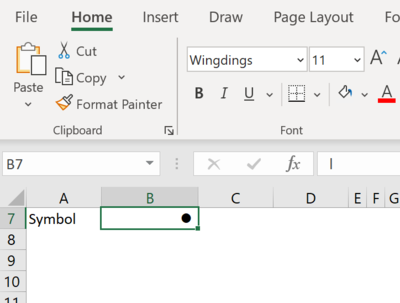6. Type in cell A8 the text label "Min" and in cell A9 the text label "Max".
7. In cell B8 type the following formula:
= MIN(B12:B161)
8. In cell B9 type the following formula:
= MAXB12:B161)
The Excel sheet should look like this: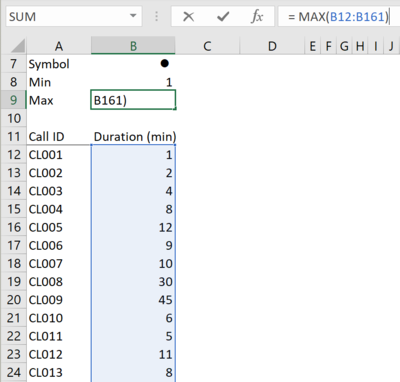9. Type in cell D8 the text label "Call duration (Minutes)".
10. In cell E8 type the following formula:
= SEQUENCE(1,B9 - B8 + 1,B8,1)
Excel will spill 45 consecutive numbers from 1 to 45 and the Excel sheet should look like this: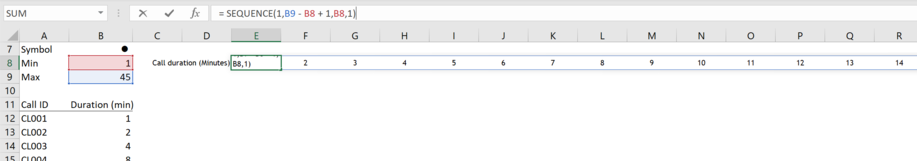11. Type in cell D7 the text label "Count of calls".
12. In cell E7 type the following formula:
= COUNTIF(\$B\$12:\$B\$161,E8#)
Excel will spill 45 numbers, the frequency of calls by duration. The Excel sheet should look like this: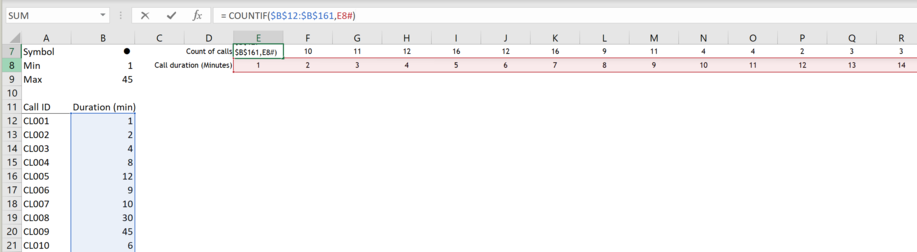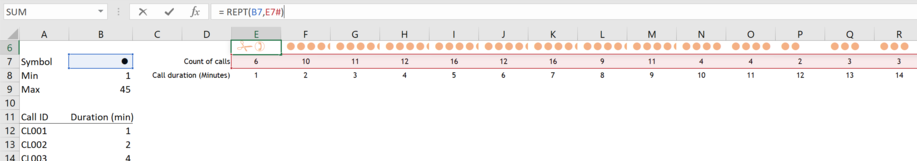17. Click the Ok button to close the Format Cells dialog box.
18. Increase the height of Row 6 so that all dots are visible.
19. Select the range E6:AW6 and set the Font Alignment to Center.
20. Select Columns E to AW and reduce their width to fit the size of the Dot.
The worksheet should look like this: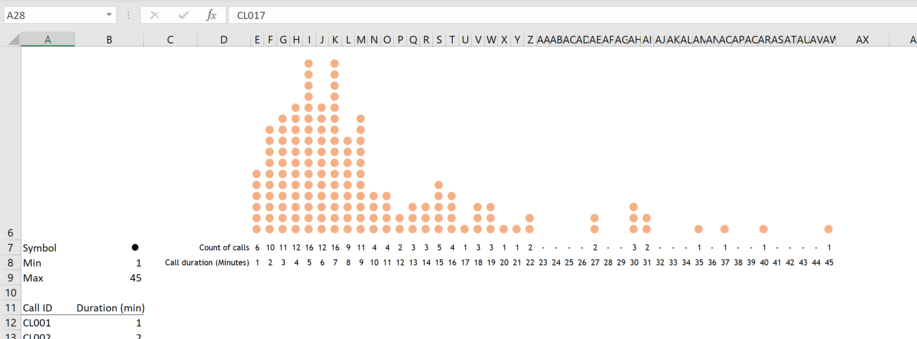Thank you for reading, and I hope you found this useful. If you have any questions, find me on Twitter and ask me anything.